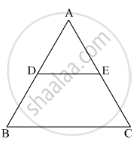# In Fig. 1, De || Bc, Ad = 1 Cm and Bd = 2 Cm. What is the Ratio of the Ar (δ Abc) to the Ar (δ Ade)? - Mathematics

Sum

In Fig. 1, DE || BC, AD = 1 cm and BD = 2 cm. What is the ratio of the ar (Δ ABC) to the ar (Δ ADE)?#### Solution

It is given that AD=1cm, BD=2 cm and DE||BC
∠A = ∠A (common angle)
By AA similarity

Ratio of area of similar triangles is equal to the square of the ratio of corresponding sides.

∴ ("ar"(Δ"ABC"))/("ar"(Δ"ADE")) = ("AB"^2)/("AD"^2)

⇒ ("ar"(Δ"ABC"))/("ar"(Δ"ADE")) = (3^2)/(1^2)

⇒ ("ar"(Δ"ABC"))/("ar"(Δ"ADE")) = (9)/(1)

Therefore, the ratio of the ar (ΔABC) : ar (ΔADE) is 9 : 1.

Concept: Ratio of Sides of Triangle
Is there an error in this question or solution?